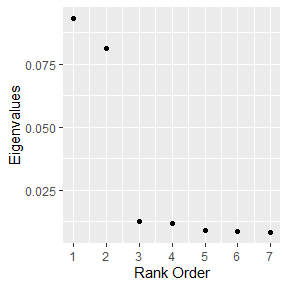# Introduction

In general, dann will struggle as unrelated variables are intermingled with informative variables. To deal with this, sub_dann projects the data onto a unique subspace and then calls dann. sub_dann is able to mitigate the use of noise variables. See section 3 of Discriminate Adaptive Nearest Neighbor Classification for details. Section 4 compares dann and sub_dann to a number of other approaches.

# Arguments

• k - The number of points in the neighborhood. Identical to k in standard k nearest neighbors. Same as dann function.
• neighborhood_size - The number of data points used to estimate a good shape for the neighborhood. Same as dann function.
• epsilon - Softening parameter. Usually has the least affect on performance. Same as dann function.
• weighted - Should the individual between class covariance matrices be weighted? FALSE corresponds to original publication.
• sphere - Type of covariance matrix to calculate.

# Example: Circle Data With Random Variables

In the below example there are 2 related variables and 5 that are unrelated. Lets see how dann, sub_dann, and dann with only the correct features perform. First, lets make a data set to work with.

 library(dann)
library(mlbench)
library(magrittr)
library(dplyr, warn.conflicts = FALSE)
library(ggplot2)

######################
# Circle data with unrelated variables
######################
set.seed(1)
train <- mlbench.circle(500, 2) %>%
tibble::as_tibble()
colnames(train)[1:3] <- c("X1", "X2", "Y")

train <- train %>%
mutate(
U1 = runif(500, -1, 1),
U2 = runif(500, -1, 1),
U3 = runif(500, -1, 1),
U4 = runif(500, -1, 1),
U5 = runif(500, -1, 1)
)

test <- mlbench.circle(500, 2) %>%
tibble::as_tibble()
colnames(test)[1:3] <- c("X1", "X2", "Y")

test <- test %>%
mutate(
U1 = runif(500, -1, 1),
U2 = runif(500, -1, 1),
U3 = runif(500, -1, 1),
U4 = runif(500, -1, 1),
U5 = runif(500, -1, 1)
)

To use with the dann package, data needs to be in matrices instead of dataframes.

 xTrain <- train %>%
select(X1, X2, U1, U2, U3, U4, U5) %>%
as.matrix()

yTrain <- train %>%
pull(Y) %>%
as.numeric() %>%
as.vector()

xTest <- test %>%
select(X1, X2, U1, U2, U3, U4, U5) %>%
as.matrix()

yTest <- test %>%
pull(Y) %>%
as.numeric() %>%
as.vector()

As expected, dann is not performant.

 dannPreds <- dann(xTrain = xTrain, yTrain = yTrain, xTest = xTest,
k = 3, neighborhood_size = 50, epsilon = 1, probability = FALSE)
mean(dannPreds == yTest) # Not a good model
##  0.668

Moving on to sub_dann, the dimension of the subspace should be chosen based on the number of large eigenvalues. The graph suggests 2 is good (the correct answer).

 graph_eigenvalues(xTrain = xTrain, yTrain = yTrain,
neighborhood_size = 50, weighted = FALSE, sphere = "mcd")While continuing to use unrelated variables, sub_dann did much better than dann.

 subDannPreds <- sub_dann(xTrain = xTrain, yTrain = yTrain, xTest = xTest,
k = 3, neighborhood_size = 50, epsilon = 1,
probability = FALSE,
weighted = FALSE, sphere = "mcd", numDim = 2)
mean(subDannPreds == yTest) # sub_dan does much better when unrelated variables are present.
##  0.882

As an upper bound on performance for this A.I. approach, lets try dann using only the informative variables. There is some improvement to be had.

 variableSelectionDann <- dann(xTrain = xTrain[, 1:2], yTrain = yTrain, xTest = xTest[, 1:2],
k = 3, neighborhood_size = 50, epsilon = 1, probability = FALSE)

mean(variableSelectionDann == yTest) # Best model found when only true predictors are used.
##  0.944

Overall, dann with the correct variables did better than sub_dann. But in practice, it is usually unknown which variables are informative. The best course of action is to find the best set of variables. But due to project timelines, this is not always an option. subb_dann is an way to gain some level of performance with minimal work.